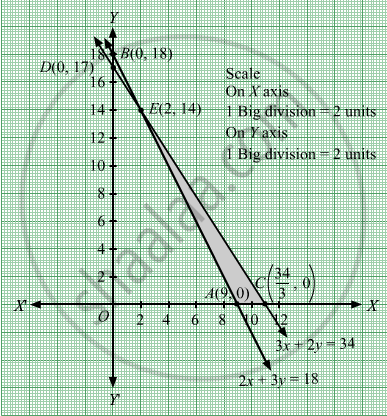# Maximize Z = 50x + 30y Subject to 2 X + Y ≤ 18 3 X + 2 Y ≤ 34 X , Y ≥ 0 - Mathematics

Sum

Maximize Z = 50x + 30y
Subject to

$2x + y \leq 18$
$3x + 2y \leq 34$
$x, y \geq 0$

#### Solution

First, we will convert the given inequations into equations, we obtain the following equations:
2x y = 18, 3x + 2y = 34

Region represented by 2x ≥ 18:
The line 2x y = 18 meets the coordinate axes at A(9, 0) and B(0, 18) respectively. By joining these points we obtain the line 2x y = 18.
Clearly (0,0) does not satisfies the inequation 2x ≥ 18. So,the region in xy plane which does not contain the origin represents the solution set of the inequation 2x ≥ 18.

Region represented by 3x + 2y ≤ 34:
The line 3x + 2y = 34 meets the coordinate axes at

$C\left( \frac{34}{3}, 0 \right)$  and D(0, 17) respectively.
By joining these points we obtain the line 3x + 2y= 34.
Clearly (0,0) satisfies the inequation 3x + 2y ≤ 34. So,the region containing the origin
represents the solution set of the inequation 3x + 2y ≤ 34.

The corner points of the feasible region are A(9, 0),

$C\left( \frac{34}{3}, 0 \right)$  and E(2, 14).The values of Z at these corner points are as follows.

Corner point Z = 50x + 30y
A(9, 0) 50 × 9 + 3 × 0 = 450
$C\left( \frac{34}{3}, 0 \right)$ 50 x $\frac{34}{3}$ + 30 x 0= $\frac{1700}{3}$
E(2, 14)
 50 × 2 + 30 × 14 = 520

Therefore, the maximum value of Z is

$\frac{1700}{3}\text{ at the point } \left( \frac{34}{3}, 0 \right)$ .Hence, x =
$\frac{34}{3}$  and y =0 is the optimal solution of the given LPP.
Thus, the optimal value of Z is $\frac{1700}{3}$ .
Concept: Graphical Method of Solving Linear Programming Problems
Is there an error in this question or solution?

#### APPEARS IN

RD Sharma Class 12 Maths
Chapter 30 Linear programming
Exercise 30.2 | Q 4 | Page 32